# Solving the Weight Puzzle: Explaining Why and How

• Tapias5000

#### Tapias5000

Homework Statement
The uniform bar is bent in the form of a parabola and has a weight per unit length of 6 lb>ft. parabola and has a weight per unit length of 6 lb>ft. Determine the reactions at the fixed support A.
Relevant Equations
## dL=\sqrt{\left(\frac{dx}{dy}\right)^2+1}dy ##
## \overline{x}=\frac{\int _{ }^{ }\tilde{x}dL}{\int _{ }^{ }dL} ##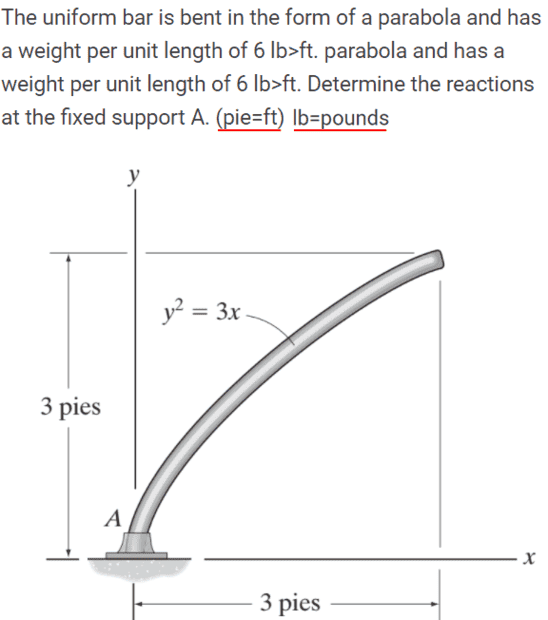My solution is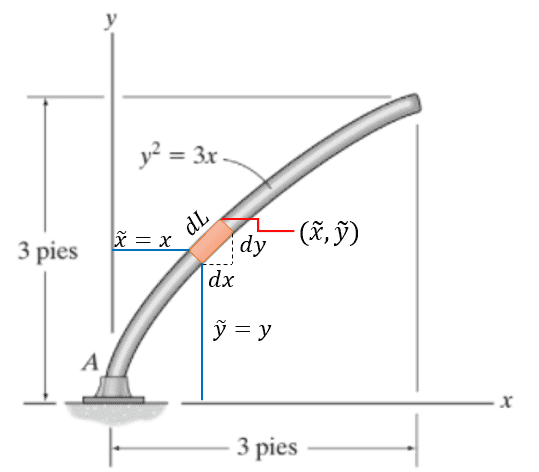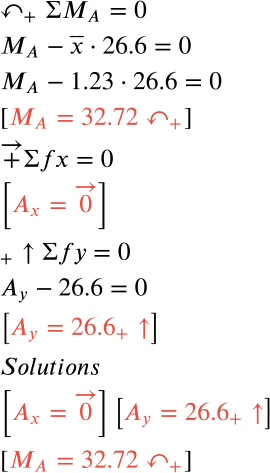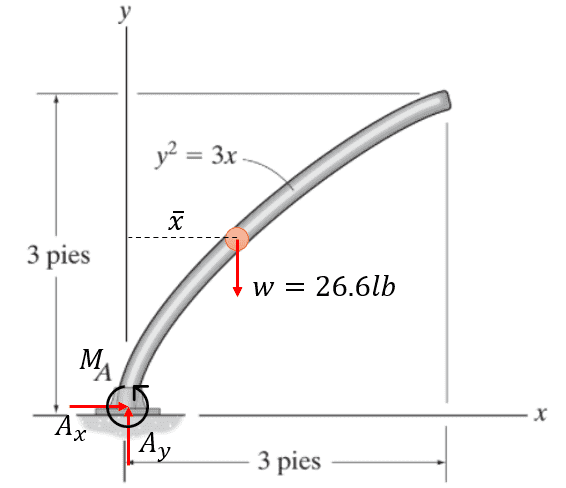However can someone explain in detail why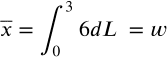will be equal to the weight? and why does it have to be multiplied by 6?